# Math test

In class 6.A are 26 students, and the teacher had managed to give tests to 13 students. 13 testes he gave in 6.5 min. How many pupils still haven't test? How long will it take the teacher to give tests to 43 students?

n =  13
t =  21.5 min

### Step-by-step explanation:

$n=26-13=13$Did you find an error or inaccuracy? Feel free to write us. Thank you!## Related math problems and questions:

• 9.A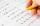9.A to attend more than 20 students but fewer than 40 students. A third of the pupils wrote a math test to mark 1, the sixth to mark 2, the ninth to mark 3. No one gets mark 4. How many students of class 9.A wrote a test to mark 5?
• Math classificationIn 3A class are 27 students. One-third got a B in math, and the rest got an A. How many students received a B in math?
• A teacher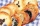A teacher baked 5 dozen cookies for the 16 students in her class. If the teacher divided the cookies equally among her students, how many cookies did she give each student?
• Youth trackYouth track from Hronská Dúbrava to Banská Štiavnica which announced cancellation attracted considerable media attention and public opposition, has cost 6.3 euro per capita and revenue 13 cents per capita. Calculate the size of subsidies to a trip group o
• Report 2A School reports students to teacher ratio of 6:1. If there are 45 teachers in the School, how many students are there?
• Students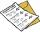The class has 22 students. 2 of which have assessments of 2. The count of assessment of 1 is three times more than the count of students with an assessment of 3. How many students have an assessment of 1, and how many students have an assessment of 1.
• Word problem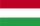348 students were on holiday in Hungary. 133 bought pizza, chips 28 pupils, 25 pupils bought soda, butter 26 pupils, 15 pupils fruits, vegetables 29 students. How much pay for all the food, if every meal cost 5.3 euros?
• The bar chartThe bar chart shows the results of the math test. How many% of pupils achieved approximately better results than the average of the class? Diagram: 1-10 pupils, 2-4 pupils, 3-6 pupils, 4-2 pupils, 5-4 pupils. (lowest result are better)
• Class alphabet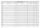All 29 pupils in the class are written in a class by alphabet. The number of pupils enrolled before Paul is three times higher than the number of pupils behind him. Calculate how many pupils are enrolled after Paul.
• Students at cinema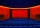All pupils from the school went to the cinema for a film performance. The cinema has 400 seats, 2 rows of 20 seats remain unoccupied. Teachers occupied 10 places. How many students were there?
• School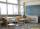27 students of 6.D class are going on a trip. They pay 9 € each. The teacher got 153 €. How many children have not paid?
• Six studentsTwo pupils painted the class in four hours. How long will it take for six pupils?
• Exhibition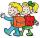The teacher paid 280 Kč for 4.A students for admission to the exhibition. How many students were at the exhibition?
• Pupils 7There are 40 pupils in a certain class. 3/5 of the class are boys. How many are girls?
• Math testStudents wrote a math test. The average number of points obtained by them was 64. Another student wrote this test for 80 points. If the teacher added his result to the original ones, the total average of all students would be 65. How many students origina
• Apples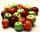Mr. Vesely give to the market his own 48 kg of apples, 40 kg from Mr. Kovar and 72 kg of Mr. Novak. Grossed a total of 1,920 CZK. How must divide income money for apples?
• School trip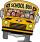School trip cost 247.2 Eur for one class (24 students). How much would a trip cost for two classes? (both classes together have 53 students)